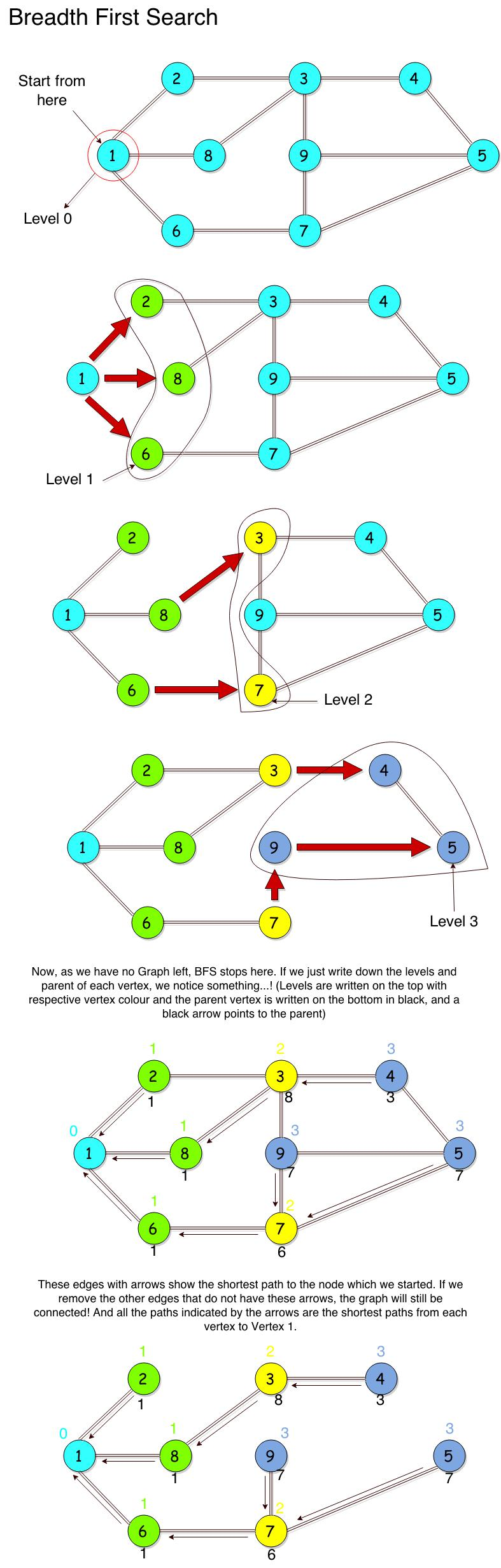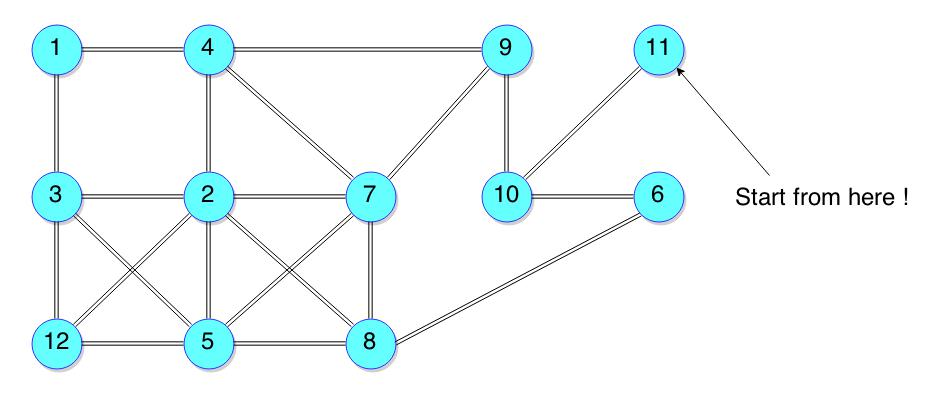101
Graph Theory - Breadth First Search
Graph Theory

In this note I will explain you one of the most widely used Graph Search Algorithms, the Breadth First Search (BFS). Once you have learned this, you have gained a new weapon in your arsenal..! You can start solving good number of Graph Theory related competitive programming questions. Not only that, but BFS has many applications in the real world. It is one of the Single Source Shortest Path Algorithms, so it is used to compute the shortest path. It is also used to solve puzzles such as the Rubick's Cube...! BFS can tell you not only the quickest way of solving the Rubick's Cube, but also the most optimal way of solving it. Pretty cool, isn't it? So, let's get started.

What we do in a BFS is a simple step-by-step process, which is -

1. Start from a vertex S. Let this vertex be at, what is called.... "Level 0".
2. Find all the other vertices that are immediately accessible from this starting vertex S, i.e., they are only a single edge away.
3. Mark these vertices to be at "Level 1".
4. There will be a challenge that you might be coming back to the same vertex due to a loop or a ring in the graph. If this happens your BFS will take time. So, you will go only to those edges who do not have a Level set to some value.
5. Mark which is the parent vertex of the current vertex your are at, i.e., the vertex from which you accessed the current vertex. Do this for all the vertices at Level 1.
6. Now, find all those vertices that are a single edge away from all the vertices which are at "Level 1". These new set of vertices will be at "Level 2".
7. Repeat this process untill you run out of graph.

This might sound heavy... Well atleast it would sound heavy to me if I heard it for the first time...! Many questions may pop-up in your mind, like, "How are we gonna do all that...?!". Well, for now, focus on the concept, we will talk about the code later. And remember, we are talking about an Undirected Graph here. We will talk about Directed Graphs later. To understand the above stated steps, examine the picture below -As we can see how step-by-step we explore the vertices adjacent to a vertex and mark their levels. If you have noticed, whenever there were two ways of accessing the same vertex from multiple vertices of the same Level, i.e., in the diagram, Vertex 3 was accessible from Vertex 2 and Vertex 8, we preferred its parent to be Vertex 8, the larger number. Why is that so... We will learn that in a short moment. The concepts that I wish to emphasize from the above picture are, how BFS can tell you the shortest path from a given vertex to all the other vertices and the number of edges or the "length of the path", a more familiar term, would be the Level of that Vertex itself. This is a very important feature of the BFS, you will understand this more clearly when I explain it with the help of an example in a different post.

Now, having got some knowledge about the BFS it is a good thing to exercise on this topic to really get the flow going. Try implementing BFS on the Graph given. All you have to do is to implement the step-by-step process and get that final figure which I got above. And make sure you label the Levels and Parents for each vertex in the end.Now, we come to the code part of the Breadth First Search, in C. The vertices are structures that have hold an integer and a pointer to the next node, just as for any linked list. Coming back to our BFS discussion, the level of each vertex is stored in a separate array and so is the case for parent of each vertex. The three arrays are initialized to appropriate values. Now recall our step-by-step process that was stated earlier. Try to implement that in our code. Take a while to think how we would do that. If you could code it, you are marvelous...! If not, don't fret, I put up my code below.

void breadth_first_search(struct node * list[], int vertices, int parent[], int level[])
{
struct node * temp;
int i, par, lev, flag = 1;
//'lev' represents the level to be assigned
//'par' represents the parent to be assigned
//'flag' used to indicate if graph is exhausted

lev = 0;
level = lev;
/* We start from node - 1
* So, Node - 1 is at level 0
* All immediate neighbours are at
* level 1 and so on.
*/

while (flag) {
flag = 0;
for (i = 1; i <= vertices; ++i) {
if (level[i] == lev) {
flag = 1;
temp = list[i];
par = i;

while (temp != NULL) {
if (level[temp->val] != -1) {
temp = temp->next;
continue;
}

level[temp->val] = lev + 1;
parent[temp->val] = par;
temp = temp->next;
}
}
}

++lev;
}
}


To test the algorithm, you can use the example depicted in the picture so that you can compare the results easily. In the code I put above, I took Vertex 1 as the starting vertex. You could change this by passing a parameter, 'startVertex', which would be an integer and modify the initializations accordingly. Now, there is an important point here, which is, when we insert a vertex into the Adjacency List, we follow Head Insertion to get O(1) Insertion. What happens due to this is that, the Linked List will have numbers in the descending order. So, when we do BFS for adjacent vertices of a given node, the Vertex with the higher number is met first. And then we explore starting by that higher numbered Vertex. This is why whenever we had a choice in approaching a vertex, we preferred approaching from the vertex which had the bigger number, why...? Due to Head Insertion...!

This is the overview of Breadth First Search Algorithm. It works perfectly for a Directed Graph too. Only the input Adjacency List would change for that. It has a computational complexity of O(|V| + |E|), which is pretty fast. But why O(|V| + |E|)...? If you think about the algorithm a little bit, we cover all the |V| vertices level-by-level, checking all the |E| edges twice (for an Undirected Graph). Once the level is set for a subset of vertices we don't visit them again. Put an itty-bitty thought into it and I'm sure you'll get the idea...! We can use BFS in the following scenarios -

• Shortest Path or Quickest Path (if all edges have equal weight).
• Finding the Length of Shortest Path between two Vertices.

I hope my note has helped in learning the BFS. If it did, let me know by commenting...! With the knowledge of BFS you can start solving Graph Theory related problems. It really depends on your logic how you will apply the BFS to the given problem. And there is only one way to develop it... Practice...! So, keep practicing... Happy Coding...! ☺

Sources and Resources -